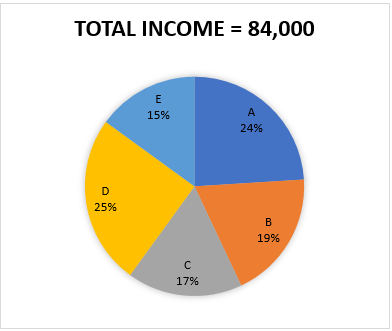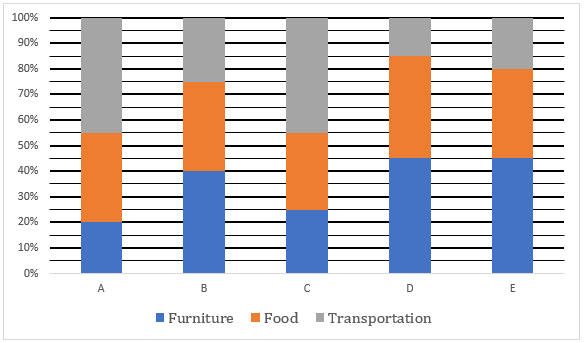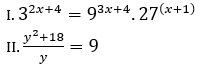### SBI PO Quantitative Aptitude Quiz

The questions asked in the quantitative aptitude section are calculative and very time-consuming. But once dealt with proper strategy, speed, and accuracy, this section can get you the maximum marks in the examination. Following is the Quantitative Aptitude quiz to help you practice with the best of latest pattern questions

Directions (1-5): Pie-chart given bellows shows the income of five different persons and bar graph shows the percentage distribution of their income on different things. Study the question carefully and answer them.Q1. Who among the following spend maximum amount on food?

D
E
B
C
A
Solution:Alternate,
By seeing the chart and graph it can be easily concluded that ‘D’ spend maximum amount on food as he has maximum income among five persons and he spend maximum on food as compare to others

Q2. Amount spend by ‘E’ on furniture is what percent more than amount spend by ‘D’ on Transportation?
70%
45%
80%
65%
60%

Q3. Find the average amount spend by A, B and C on furniture?
4622
4626
4262
4266
4662

Q4. ‘D’ buy only three type of food X, Y and Z and amount spend on buying X, Y and Z is in the ratio 5 : 7 : 8. What is the difference between amount spend on buying Z type food to amount spend on buying X type food.
2520
1680
8400
1260
2100

Q5. Find the ratio of amount spend by ‘A’ and ‘B’ together on food to the amount spend by ‘C’ and ‘D’ together on furniture?
295 : 277
277 : 295
310 : 301
301 : 305
301 : 310

Directions (6-10): Solve the given quadratic equations and mark the correct option based on your answer—

Q6.x > y
x ≥ y
x < y
x ≤ y
x = y or no relation can be established between x and y.

Q7.x > y
x ≥ y
x < y
x ≤ y
x = y or no relation can be established between x and y.

Q8.x > y
x ≥ y
x < y
x ≤ y
x = y or no relation can be established between x and y.

Q9.x > y
x ≥ y
x < y
x ≤ y
x = y or no relation can be established between x and y.

Q10.x > y
x ≥ y
x < y
x ≤ y
x = y or no relation can be established between x and y.

Q11. A man buys ‘x’ kg of groundnuts, and their seeds weigh 60% of their total weight. The seed crushed for first time to yield oil, which is 30% of weight of seed. While the crushed waste material is treated once again & it yields 6% of oil by weight. If the amount of oil obtained at 2nd time is 25.2 kg. Find the value of x(in kg).
1300
1500
1050
1000
1200

Q12. A and B started business, where A invested his 10% savings and B invested 20% of his saving for 7 months and 10 months respectively. If B get 41 ⅔% of total profit, find the total saving of A, given that 1% of B’s savings is Rs. 500.
Rs. 100000/-
Rs. 50000/-
Rs. 200000/-
Rs. 150000/-
None of these

Q13. Rahul bought a cycle at a discount of 16⅔% on MRP. He earned half the amount of his CP by renting it for 200 days. After that he resells it at half of MRP. In this transaction he earned Rs. 200, find MRP of cycle (in Rs).
1860
2490
2400
2280
2310

Q14. In an election survey, 83 ⅓% of total voters took part in survey, 50% then claims to votes for candidate A, 10% are uncertain & rest says to vote for B. If all of them voted according to their commitments on the day of election and those who were not part of survey, voted to A and B in ratio of 2 : 1. All the people who are uncertain in survey, voted to A : B in the ratio of 1 : 4 in election. If A won by 640 votes then find total number of votes in election.
6000
5000
9000
7200
6750

Q15. An alloy has Zn : Tn : Ab in the ratio of 3 : 2 : 8. Zn is 6% impure, Tn is 4% & Ab is 3.5% impure. Then find total impurity in 221 gm of alloy?
8.15 gm
9.18 gm
8.00 gm
10.95 gm
10.18 gm

You May also like to Read: